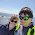## Friday, March 19, 2010

### Java interview question: Count the number of “1” bits in a byte

Frankly, I think it is quite an idiotic question. Not because it is too easy or too hard question, but because I think this question doesn’t really imply on the practical programming capabilities of the person being interviewed. Anyway, a friend of mine was asked to solve this question, so I though it might be an interest for more developers looking for a job and having to handle all sort of weird questions.
In general, there are 2 solutions for this question:
• First solution is iterating 8 times (a byte contains 8 bits). Each time the right most bit is extracted from the byte. and then the byte is shifted right one bit.
• Second solution, is a little bit less straight forward, but more efficient: Divide the number by 2, if there is a modulo, count it. Repeat this operation as long as the number we are dividing is greater than zero.
Here is how the first solution looks in Java code:
```public class GetBits {
public static int countBits(byte num)
{
int count = 0;
for (int i = 0; i < 8; i++)
{
if ((num & 1) == 1) // check if right most bit is 1
{
count++;
}
num = (byte)(num >>> 1); // shit right 1 bit, including the sign bit
}
return count;
}
```

Here is how the second solution looks like:

```public static int countBits(byte num)
{
int count = 0;
while (num > 0)
{
if (num % 2 == 1) // check if number have modulo
{
count++;
}
num /= 2; // divide the number by 2
}
return count;
}```

Note, that the first solution is a bit less efficient, because it always iterates 8 times. But, it handles both positive and negative values. The second solution will simply won’t work with negative numbers.

1.Thank you ! =)

2.I'm fairly sure your first solution doesn't work for negatives either.

3.Yeah, change this num = (byte)(num >>> 1); to this num /= 2. Otherwise this code won't work due to sign extension occurring when you shift.

4.// shit right 1 bit, including the sign bit
Think you mighta missed a letter that heh...